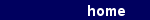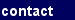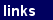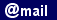The isometric illustration beside shows conventional rectangular plates and edge bars (l.) compared to the new, oblique building elements (r.). The protruding part of each edge bar can be cut off by a diagonal cut through the profile. Also among lozenge-shaped plates there can exist, as can be seen at right, such plates, which are positioned in a horizontal or vertical plane. To demonstrate this, here were chosen plates shaped like the faces of a rhombicuboctahedron. Depending on its orientation such a zonohedron of thirty faces has -- just as a dodecahedron or an icosahedron - two, three or five symmetry axes going through the centre. In this case it has been orientated in a twofold symmetry - in top view / in a plan. Indeed, there is the advantage that two horizontal symmetry axes are situated rectangular each to another. However, four of them would be even better - in order to get equivalent joining facilities in four directions. That is why all the subsequent examples are made from a zonohedron of 132 faces having four symmetry axes - or from portions of it. Due to his larger number of faces, this one here is more versatile as the basis for our building system.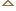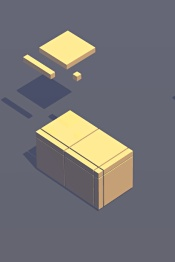#####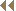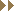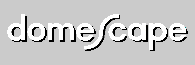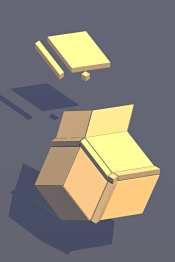©  F l o r i a n  T u c z e k  2 0 0 3 - 2 0 1 0D i s c l a i m e r(Best view  IE 6.0 1024 x 768  Pixel) S i t e  d e s i g n   b y  k u n s t k o m p l e x